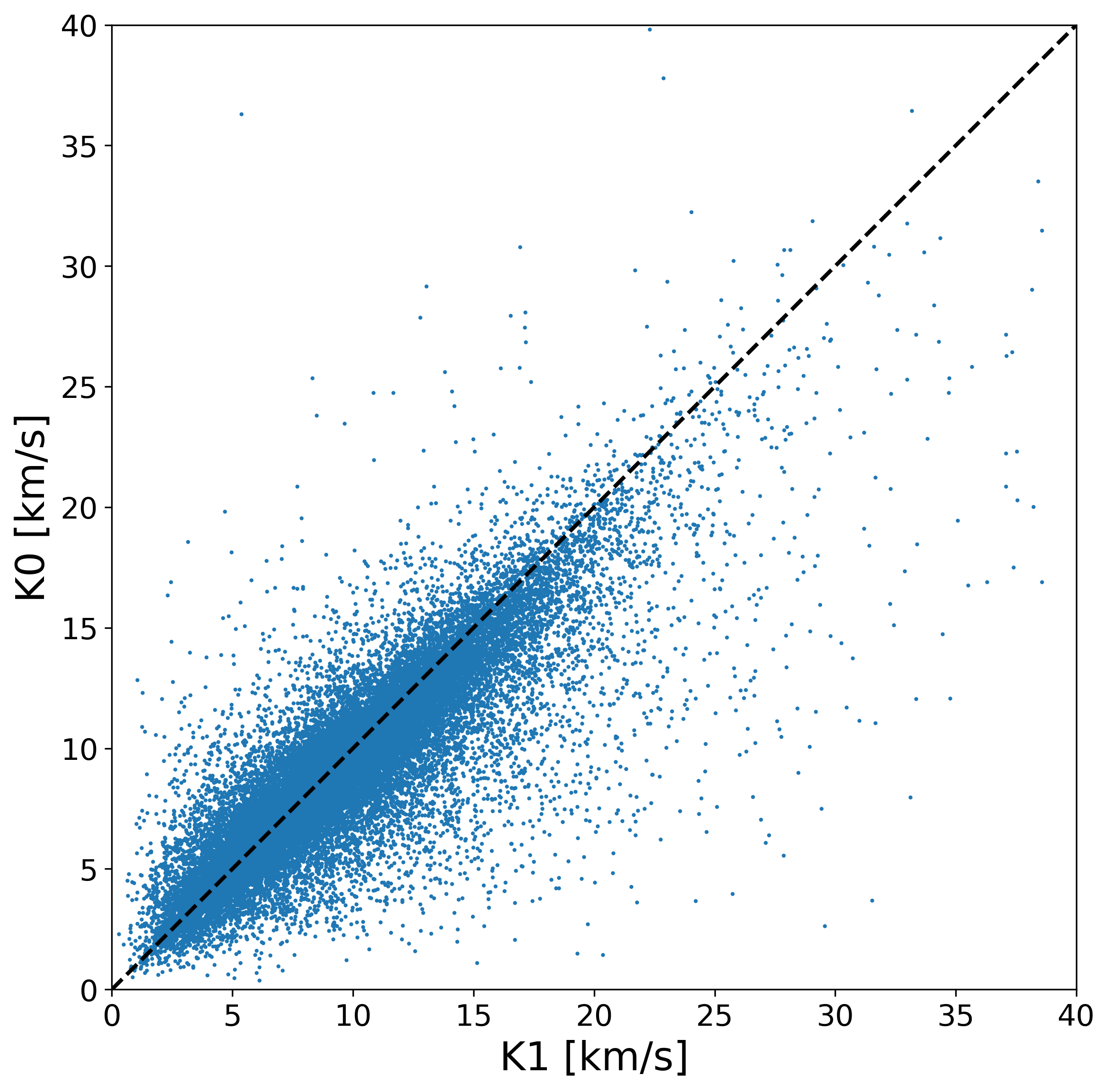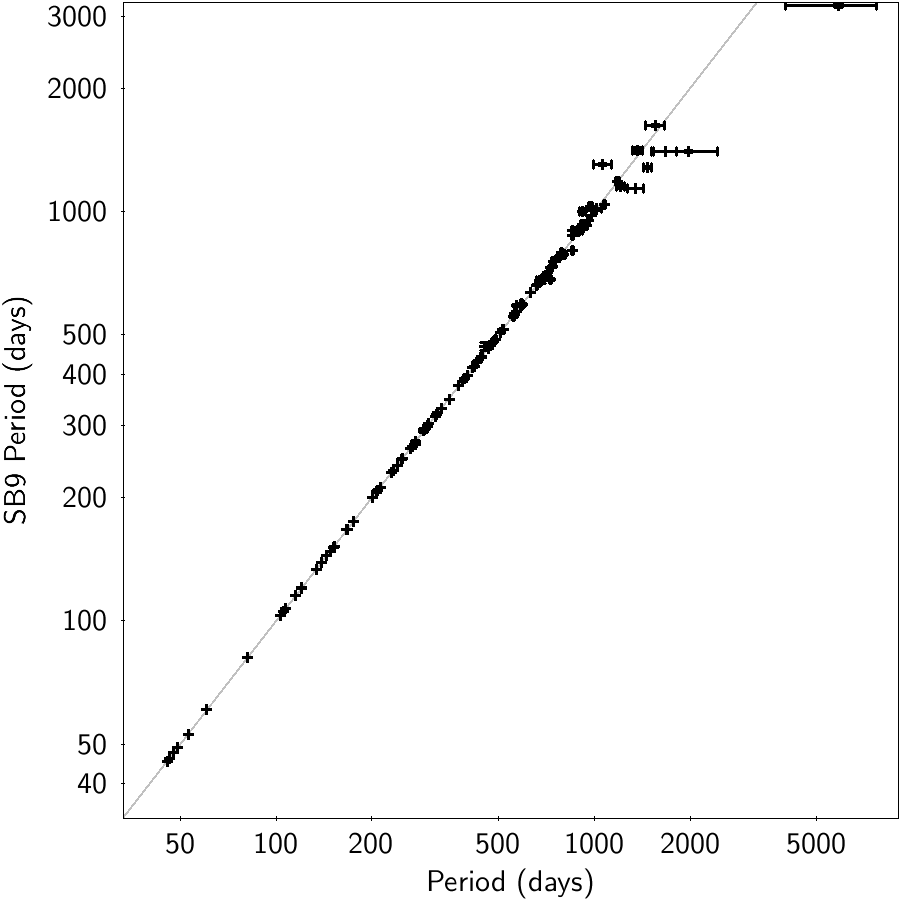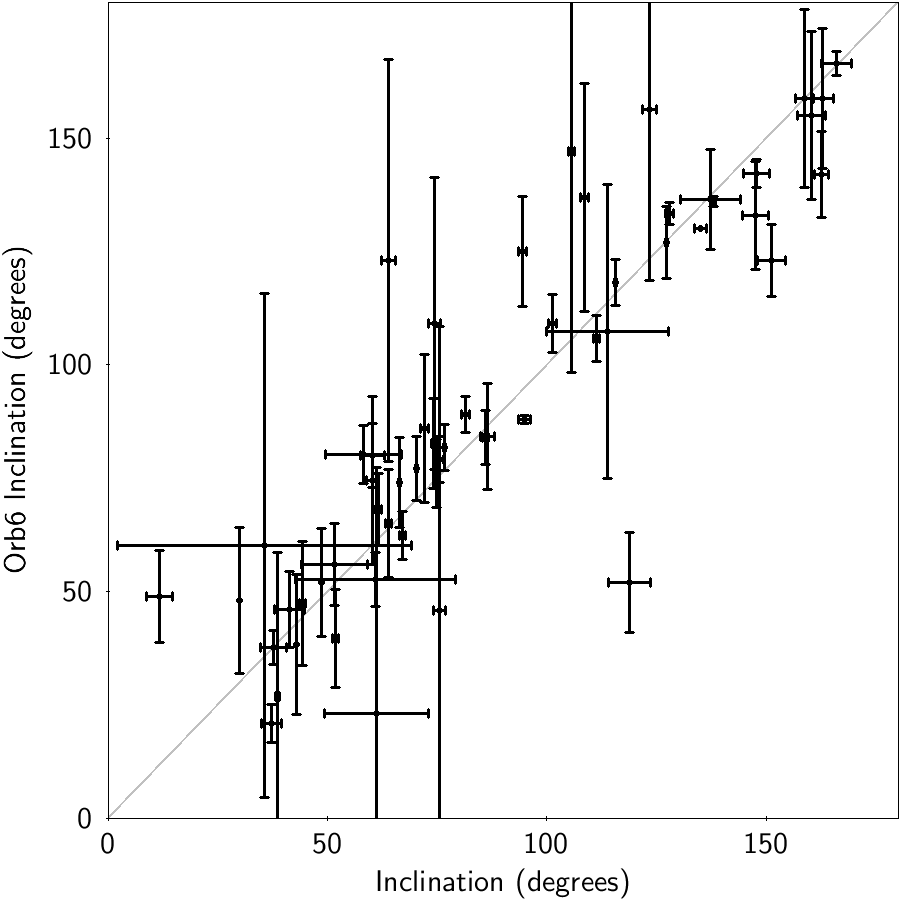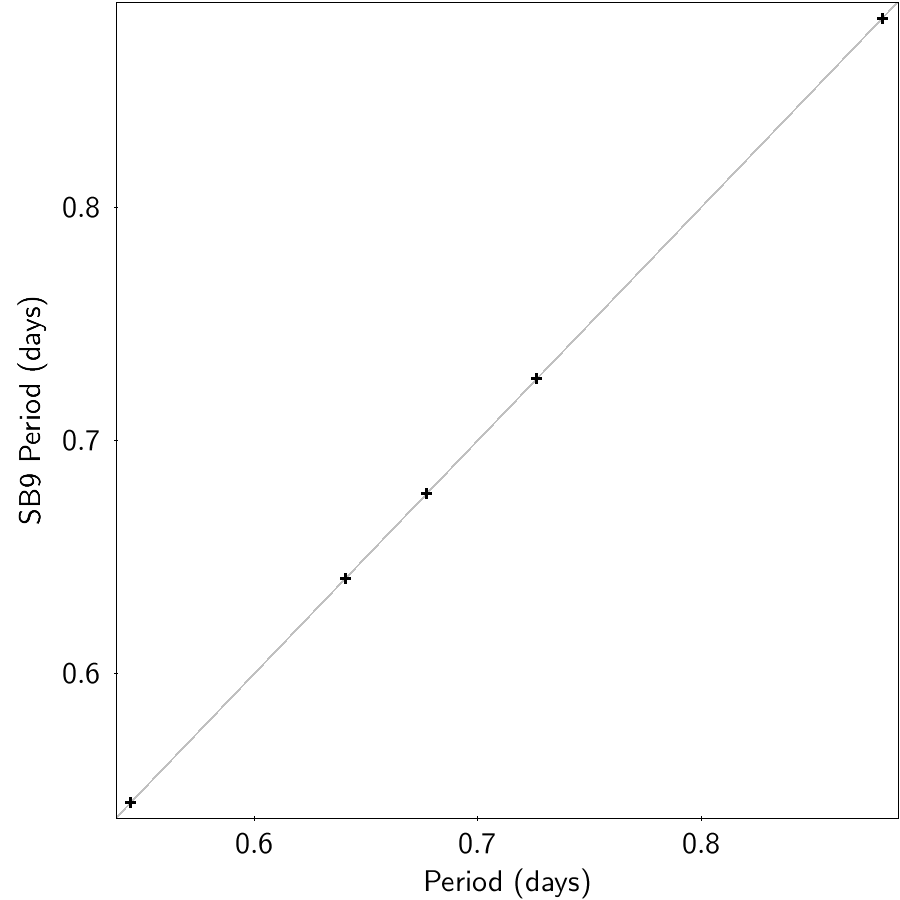# 7.7.4 Quality assessment and validation

## Verification and posterior filtering

The combined solutions have been assessed in terms of fit quality, significance of the solutions and their comparison with the parameters provided by the input solutions. Several problems were found late in the process and rejecting solutions was the only solution. Due to the different nature of the output models different criteria were used.

• AstroSpectroSB1: A set of criteria has been defined for the AstroSpectroSB1 solutions in order to accept them or not. It is presented in Equation 7.55 and involves the goodness_of_fit $F2$, the significance of the angular semi-major axis of the photocentre, the efficiency of the solution,the significance of the semi-amplitude and the difference between semi-amplitudes. The last constrain can only be applied when the spectroscopic solution provides a $K_{\mathrm{spectro}}$.

 $\displaystyle{F2_{\mathrm{AS}}}<{F2_{\mathrm{astro}}}$ (7.55) $\displaystyle\mathrm{significance}_{\alpha_{0}}>\mathrm{significance}_{\mathrm% {limit}}$ $\displaystyle 0.2<\mathrm{efficiency}\ \mathrm{OR}\ \mathrm{efficiency}=0$ $\displaystyle\mathrm{significance}_{K_{\mathrm{AS}}}\geq 5$ $\displaystyle|K_{\mathrm{AS}}-K_{\mathrm{spectro}}|\leq 5\sigma$

Where AS corresponds to a value of the combined solution, astro the value of the input astrometric binary solution and spectro the value of the input spectroscopic binary solution. The $\mathrm{significance}_{\mathrm{limit}}$ of the semi-major axis gets different values depending on the input model of the astrometric binary solution. It is the maximum value between the significance provided from the solution and a default value of each model. For StochasticAstro and OrbitalAlternative solutions the default value is 12 while for Orbital and OrbitalPoorlyConstrained is 5. $K_{\mathrm{AS}}$ is estimated using the spectroscopic part of the combined solution and its significance is estimated using an approximation on its uncertainty. A visual inspection of the fitted radial velocities led to the rejection of about 2 300 sources with an inadequate fit. Applying the above constrains $\sim$ 33 thousand combined solutions have passed to the output.

• EclipsingSpectro: Similar criteria have been applied in the result of the eclipsing and spectroscopic binaries combination in order to produce a combined solution. They are shown in Equation 7.56 where ES refer to the values of the combined EclipsingSpectro solutions and spectro the spectroscopic binary input solution. It contains limits on the compatibility of the semi-amplitude of the radial velocity fit between the input and the output, the significance of the produced semi-amplitude value and the efficiency. Finally, constrains were applied on the g_rank defined in nss_two_body_orbit-g_rank and the gmaxdist (maximum distance in phase and flux in the band)

 $\displaystyle|K_{\mathrm{ES}}-K_{\mathrm{spectro}}|\leq 5\sqrt{{e_{K_{\mathrm{% ES}}}}^{2}+{e_{K_{\mathrm{spectro}}}}^{2}+1}$ (7.56) $\displaystyle\mathrm{significance}_{K}\geq 5$ $\displaystyle\mathrm{efficiency}\geq 0.5$ $\displaystyle g\_\mathrm{rank}\geq 0.6$ $\displaystyle\mathrm{gmaxdist}\leq 0.5$

This filtering rejected most of the combinations, leaving 155 EclipsingSpectro solutions to the output.

The resulted solutions are shown in the period-eccentricity diagram presented in Figure 7.58. The two models occupy different areas as the astrometric binaries produce orbits with periods larger than $\sim 10$ days while the limit for eclipsing and spectroscopic binaries is lower. The lack of solutions with periods close to 1 year is still present. A short fraction (106) of sources have an eccentricity larger than 0.9 with the majority of them having periods above 1 000 days.

From Equation 7.7.3, the two independent T-I parts, $A,B,F,G$ for the astrometric part and $C,H$ for the spectroscopic one, can provide a comparison of parameters. The astrometric side provides a semi-major axis of the photocentre, from which a semi-amplitude $K_{0}$ can be compared to the one of the primary $K_{1}$, Figure 7.59a. The periastron argument from astrometry can also be compared to the one derived from $C/H$, Figure 7.59b. Compared to Figure 7.18, it can be seen that the input selection for the combinations used more consistent astrometric and spectroscopic orbits.Figure 7.59: Internal comparisons of the astrometric part of the AstroSpectroSB1 solutions to their spectroscopic part: semi-amplitude (a) and periastron argument (b). The convention Equation 7.59 has been applied on the astrometric periastron argument.

## Validation

After the final filtering 33 467 AstroSpectroSB1 and 155 EclipsingSpectro sources have been left. These sources have been compared with external catalogues, in order to validate the correctness of the solutions. Cross-matching with $S_{B^{9}}$: The 9th Catalogue of Spectroscopic Binary Orbits (SB9, Pourbaix et al. 2004) and Sixth Catalog of Orbits of Visual Binary Stars (Orb6, Hartkopf et al. 2001). 147 sources among the AstroSpectroSB1 have been identified in SB9 and their comparison of the orbital parameters are presented in Figures 7.60 and 7.61. In this figures the horizontal axes refer to the results of this work while the ordinate refers to the values of SB9. In all 4 plots most of the values fall in the diagonal showing good agreement. Some discrepancies are presented in the semi-amplitude of the radial velocity curve. Also there are few cases where the computed uncertainties are very large.Figure 7.60: Comparison (SB9 vs. Gaia) of period (a) and eccentricity (b) with AstroSpectroSB1 binaries found in SB9.Figure 7.61: Comparison of semi-amplitude of radial velocity (a) and systemic velocity (b) with AstroSpectroSB1 binaries found in SB9.

In the comparison with Orb6, 56 stars found to be common. Figures 7.62 and 7.63 show the comparison of period, eccentricity, inclination and semi-major axis. While the period recovery is in good agreement with the exception of 2 sources, the other orbital parameters are difficult to compare due to the large uncertainties, however they tend to fall on the 1:1 line.Figure 7.62: Comparison of period (a) and eccentricity (b) with orbital parameters of AstroSpectroSB1 binaries found in Orb6.Figure 7.63: Comparison of inclination (a) and semi-major axis (b) with orbital parameters of AstroSpectroSB1 binaries found in Orb6.

Only 5 EclipsingSpectro sources found to exist in SB9 catalog and none in Orb6. Figures 7.64 and 7.65 show the comparison of the orbital parameters between the results of this work and SB9. All EclipsingSpectro solutions where found to be circular thus no uncertainties were calculated for eccentricity. The period uncertainties are too small to be visible in the figure. The sample is too small to derive safe conclusions but the results are in agreement in all five sources with just one found to have non-circular orbit in SB9.Figure 7.64: Comparison of period (a), eccentricity (b) with EclipsingSpectro binaries found in SB9.Figure 7.65: Comparison of semi-amplitude of radial velocity (a) and systemic velocity (b) with EclipsingSpectro binaries found in SB9.